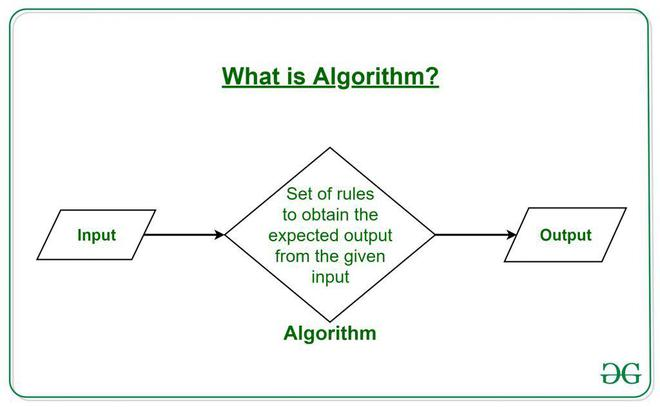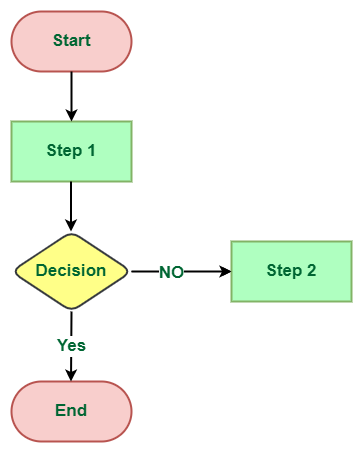Open In App

# Difference Between Algorithm and Flowchart

## What is an Algorithm?

The word Algorithm means “a process or set of rules to be followed in calculations or other problem-solving operations”. Therefore Algorithm refers to a set of rules/instructions that step-by-step define how a work is to be executed in order to get the expected results. Let’s take a look at an example for a better understanding. As a programmer, we are all aware of the Linear Search program. (Linear Search### Algorithm of linear search:

• Start from the leftmost element of arr[] and one by one compare x with each element of arr[].
• If x matches with an element, return the index.
• If x doesn’t match with any of elements, return -1.

## What is a Flowchart?

A flowchart is a graphical representation of an algorithm. Programmers often use it as a program-planning tool to solve a problem. It makes use of symbols that are connected among them to indicate the flow of information and processing. The process of drawing a flowchart for an algorithm is known as “flowcharting”. Example: Draw a flowchart to input two numbers from the user and display the largest of two numbers